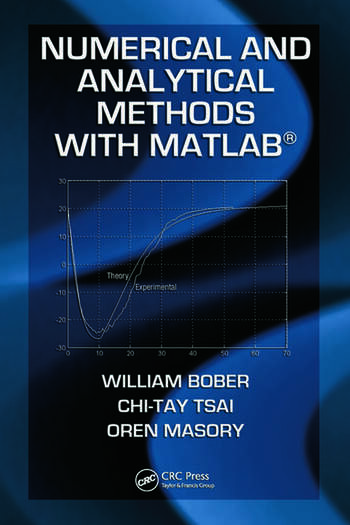# Numerical and Analytical Methods with MATLAB

## 1st Edition

William Bober, Chi-Tay Tsai, Oren Masory

CRC Press
Published August 11, 2009
Textbook - 488 Pages - 267 B/W Illustrations
ISBN 9781420093568 - CAT# 93568
Series: Applied and Computational Mechanics

For Instructors Request Inspection Copy

was \$150.00

USD\$120.00

SAVE ~\$30.00

FREE Standard Shipping!

## Preview

### Summary

Numerical and Analytical Methods with MATLAB® presents extensive coverage of the MATLAB programming language for engineers. It demonstrates how the built-in functions of MATLAB can be used to solve systems of linear equations, ODEs, roots of transcendental equations, statistical problems, optimization problems, control systems problems, and stress analysis problems. These built-in functions are essentially black boxes to students. By combining MATLAB with basic numerical and analytical techniques, the mystery of what these black boxes might contain is somewhat alleviated.

This classroom-tested text first reviews the essentials involved in writing computer programs as well as fundamental aspects of MATLAB. It next explains how matrices can solve problems of linear equations, how to obtain the roots of algebraic and transcendental equations, how to evaluate integrals, and how to solve various ODEs. After exploring the features of Simulink, the book discusses curve fitting, optimization problems, and PDE problems, such as the vibrating string, unsteady heat conduction, and sound waves. The focus then shifts to the solution of engineering problems via iteration procedures, differential equations via Laplace transforms, and stress analysis problems via the finite element method. The final chapter examines control systems theory, including the design of single-input single-output (SISO) systems.

Two Courses in One Textbook
The first six chapters are appropriate for a lower level course at the sophomore level. The remaining chapters are ideal for a course at the senior undergraduate or first-year graduate level. Most of the chapters contain projects that require students to write a computer program in MATLAB that produces tables, graphs, or both. Many sample MATLAB programs (scripts) in the text provide guidance on completing these projects.

#### Instructors

We provide complimentary e-inspection copies of primary textbooks to instructors considering our books for course adoption.